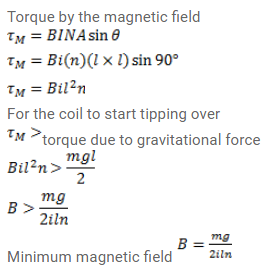# A square coil of edge I having n turns carries a current i.

Question:

A square coil of edge I having n turns carries a current i. It is kept on a smooth horizontal plate. A uniform magnetic field B exists in a directional parallel to an edge. The total mass of the coil is $M$. What should be the minimum value of $B$ for which the coil will start tipping over?

Solution: